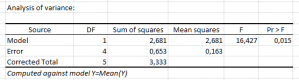# Ordinary Least Squares regression (OLS)

Ordinary Least Squares regression, often called linear regression, is available in Excel using the XLSTAT add-on statistical software.## Equations for the Ordinary Least Squares regression

Ordinary Least Squares regression (OLS) is more commonly named linear regression (simple or multiple depending on the number of explanatory variables).

In the case of a model with p explanatory variables, the OLS regression model writes:

Y = β0 + Σj=1..p βjXj + ε

where Y is the dependent variable, β0, is the intercept of the model, X j corresponds to the jth explanatory variable of the model (j= 1 to p), and e is the random error with expectation 0 and variance σ².

In the case where there are n observations, the estimation of the predicted value of the dependent variable Y for the ith observation is given by:

yi = β0 + Σj=1..p βjXij

The OLS method corresponds to minimizing the sum of square differences between the observed and predicted values. This minimization leads to the following estimators of the parameters of the model:

[β = (X’DX)-1 X’ Dy σ² = 1/(W –p*) Σi=1..n wi(yi - yi)] where β is the vector of the estimators of the βi parameters, X is the matrix of the explanatory variables preceded by a vector of 1s, y is the vector of the n observed values of the dependent variable, p* is the number of explanatory variables to which we add 1 if the intercept is not fixed, wi is the weight of the ith observation, and W is the sum of the wi weights, and D is a matrix with the wi weights on its diagonal.

The vector of the predicted values can be written as follows:

y = X (X’ DX)-1 X’Dy

## Limitation of the Ordinary Least Squares regression

The limitations of the OLS regression come from the constraint of the inversion of the X’X matrix: it is required that the rank of the matrix is p+1, and some numerical problems may arise if the matrix is not well behaved. XLSTAT uses algorithms due to Dempster (1969) that allow circumventing these two issues: if the matrix rank equals q where q is strictly lower than p+1, some variables are removed from the model, either because they are constant or because they belong to a block of collinear variables.

## Variable selection in the OLS regression

An automatic selection of the variables is performed if the user selects a too high number of variables compared to the number of observations. The theoretical limit is n-1, as with greater values the X’X matrix becomes non-invertible.

The deleting of some of the variables may however not be optimal: in some cases we might not add a variable to the model because it is almost collinear to some other variables or to a block of variables, but it might be that it would be more relevant to remove a variable that is already in the model and to the new variable.

For that reason, and also in order to handle the cases where there a lot of explanatory variables, other methods have been developed.

## Prediction

Linear regression is often use to predict outputs' values for new samples. XLSTAT enable you to characterize the quality of the model for prediction before you go ahaed and use it for predictive use.### analice sus datos con xlstat

prueba gratuita de 14 días

Incluido en